cancel
Showing results for
Did you mean: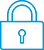It has been a while since anyone has replied. Simply ask a new question if you would like to start the discussion again.New member
2 1 0 0
Message 1 of 4
914
Flag Post

Solved!

# Hp Prime displays incorrects values for function with cube rootHP Recommended
Hp Prime

When I see the table of values for this function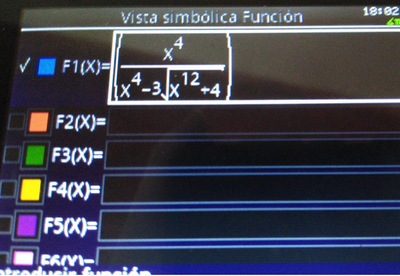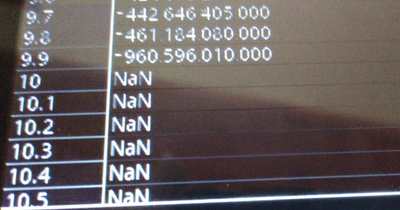It says that the function is not defined for values higher than 10. When I copy-paste and replace the X with 11, however, I do get a result.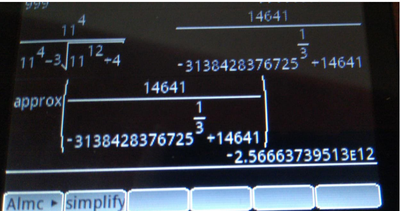Also, trying different values that appeared defined in the table, gives different results: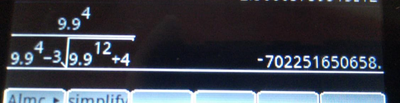What is going? What is the real result?

Tags (1)
1 ACCEPTED SOLUTION

Accepted Solutions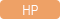Level 6
252 251 42 78
Message 4 of 4
Flag PostHP Recommended

Hello,

Ok, got it...

X^12 when X is 10 is 1e12... 1e12+4 is STILL 1e12 as the calculator only has 12 digit accuracy. take the cube root and get 1e4. X^4 with X=10 = 1e4, 1e4-1e4 = 0, you end up doing a divide by 0... which is undefined....

You are hitting the limit of what floating point arithmetic can do with 12 digit precision in decimal.

Now, let us move to the CAS side of the equation.

The CAS does NOT use decimal arithmetics, it uses binary arithmetics. It uses 44 bit of precision but only 11 bits of exponent.

This means that, to simplify, the CAS has a little bit more precision (14 digits from memory), but with a lesser range (the exponent is limited to + or - 308 vs the + or - 500 of the home/decimal system)...

As a result, this operation will still work in the CAS. In CAS, the problem will only apear a little bit later.

In cas, try 1e16+4 ENTER Ans-1e16 and you will get 0. Try 1e12+4 ENTER Ans-1e12 and you will get 4...

Cyrille

I am an HP Employee
Tags (1)
3 REPLIES 3Level 6
252 251 42 78
Message 2 of 4
Flag PostHP Recommended

Hello,

This is definitely very strange. I get -0.5 here, which is the correct result.You can tell that it is correct as, when X gets big, the +4 in the X^12 becomes negligeable, so the X^12+4 is roughtly equal to X^12. The 3rd root of X^12 is X^4 (for X real). so you end up with the expression (at the bottom) X^4-3*X^4 = -2X^4.

You can simplify the top and bottom of your fraction and you get 1/-2 or 0.5 as the limit for your function when X gets big.

This is what I am getting on my prime.

What version of the FW are you using?

Cyrille

I am an HP EmployeeNew member
2 1 0 0
Message 3 of 4
Flag PostHP Recommended

I think you misread the 3 from the cube root as a factor, the expression in the denominator is not x^4 - 3 * (x^4 +4) ^1/3 but just x^4 - (x^4 +4) ^1/3. The limit when x tends to infinity should be negative infinity.

My software version is 2.1.14181 (2018 10 16)

Hardware version: DLevel 6
252 251 42 78
Message 4 of 4
Flag PostHP Recommended

Hello,

Ok, got it...

X^12 when X is 10 is 1e12... 1e12+4 is STILL 1e12 as the calculator only has 12 digit accuracy. take the cube root and get 1e4. X^4 with X=10 = 1e4, 1e4-1e4 = 0, you end up doing a divide by 0... which is undefined....

You are hitting the limit of what floating point arithmetic can do with 12 digit precision in decimal.

Now, let us move to the CAS side of the equation.

The CAS does NOT use decimal arithmetics, it uses binary arithmetics. It uses 44 bit of precision but only 11 bits of exponent.

This means that, to simplify, the CAS has a little bit more precision (14 digits from memory), but with a lesser range (the exponent is limited to + or - 308 vs the + or - 500 of the home/decimal system)...

As a result, this operation will still work in the CAS. In CAS, the problem will only apear a little bit later.

In cas, try 1e16+4 ENTER Ans-1e16 and you will get 0. Try 1e12+4 ENTER Ans-1e12 and you will get 4...

Cyrille

I am an HP Employee
Tags (1)
† The opinions expressed above are the personal opinions of the authors, not of HP. By using this site, you accept the Terms of Use and Rules of Participation